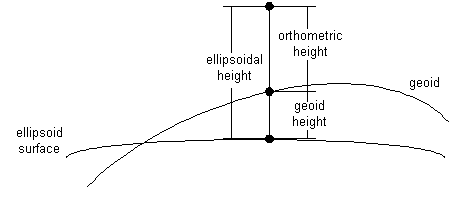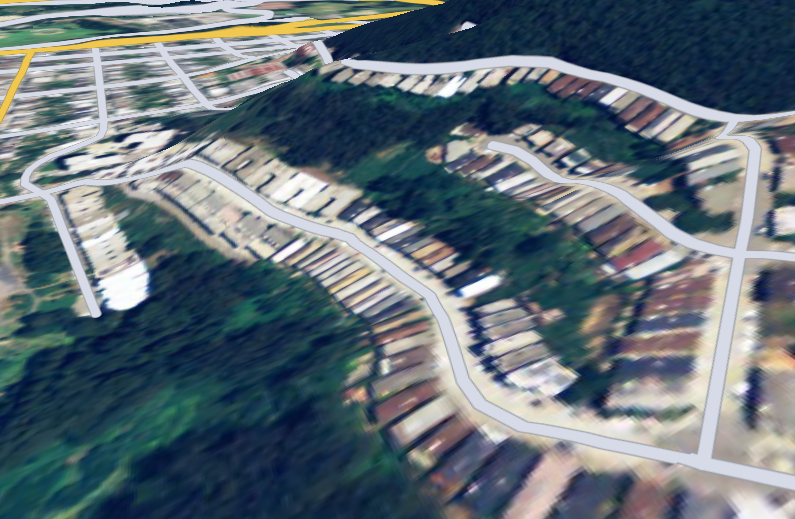## What is geodesy?

Geodesy is the scientific discipline that deals with the measurement and representation of the earth.

Many mathematical models have been proposed to approximate the shape of the earth, ranging from flat-earth models to spherical approximations, ellipsoid, and geoid. Spherical and ellipsoidal approximations are used because of their mathematical simplicity. A more exact approximation is the earth’s geoid, a hypothetical surface that coincides with the earth’s mean sea level. Although the earth’s geoid is considerably smoother than the actual surface of the earth, it’s still highly irregular. That’s why it’s more difficult to represent and use in computations.

Note that there are many ellipsoid models — for example WGS84 and NAD83-- and geoid models — for example EGM96 and NAVD88 — in use around the world. The following sections describe what references LuciadRIA supports and how you can use them.

## What is a geodetic datum?

A geodetic datum is a reference from which position measurements are made.

A horizontal datum is a known and constant surface on which the positions of points can be precisely expressed. Because of their relative simplicity, ellipsoids are often used as the basis for horizontal datums.

A vertical datum is an extra vertical reference for expressing the elevation of points. It’s typically based on geoid or ellipsoid models.

Geodetic datums provide a basis for coordinate reference systems. For example, a geodetic datum based on an ellipsoid model of the earth’s surface allows to define geodetic lon-lat-height coordinates. The height coordinate may for example be computed relative to the ellipsoid on which the lon-lat coordinates are defined (ellipsoidal height), or relative to a geoid model (orthometric height), as shown in Figure 1, “Ellipsoidal versus orthometric heights”.

Geodetic datums and the coordinate systems based on them are widely used in surveying, mapping, and navigation.Figure 1. Ellipsoidal versus orthometric heights

Many datums are in use today, as the basis for even more coordinate reference systems. Because referencing geodetic coordinates to the wrong datum can result in position errors of hundreds of meters, you need to be careful when converting between coordinates defined with respect to different datums. You can find more information on coordinate transformations in Transforming coordinates between geographic references.

LuciadRIA implicitly supports geodetic datums through the various coordinate reference systems that it supports. The API reference documentation provides all the details in the modules `reference`, `transformation` and `geodesy`.

## Working with coordinate references

When you create a model, you need a `CoordinateReference`. A `CoordinateReference` specifies the coordinate reference system used for locating the model data on earth. You must link a model to a `CoordinateReference` to define the objects in the model in a uniform way.

The `reference` module provides a `ReferenceProvider` that you can use to get a suitable `CoordinateReference`, based on an EPSG code.

Program: Creating a reference illustrates how you get a WGS84 reference from the reference factory using its `EPSG:4326` code.

Program: Creating a reference (from `samples/common/LayerFactory.ts`)
``const tileSetReference = getReference("EPSG:4326");``

`ReferenceProvider` supports some commonly used references by default. See the reference documentation of the `isValidReferenceIdentifier(referenceIdentifier)` method for a list of those references.

You can also parse a reference formatted in Well-Known Text (WKT). Use the `parseWellKnownText` method for that.

## Working with vertical references

As mentioned in What is a geodetic datum?, a `CoordinateReference` has both a horizontal datum and a vertical datum.

The vertical datum in the `CoordinateReference` generated by Program: Creating a reference has a vertical reference that is defined relative to Above Mean Sea Level (AMSL). This has effect on the z-coordinate of the shapes in your model. A z-coordinate equal to 0 places your shape on the Mean Sea Level reference plane.

The `ReferenceProvider` class also allows you to create references that with a vertical reference defined above the terrain contained in the view:

Program: Create a height above terrain `CoordinateReference` using the factory methods from the `ReferenceProvider` module
``const reference = getHeightAboveTerrainReference("EPSG:4326");``

Program: Create a height above terrain `CoordinateReference` shows you how to create a `CoordinateReference` that’s defined above the terrain’s surface. This has an effect on the z-coordinates of the shapes in your model. In a height above terrain reference, a z-coordinate equal to 0 places your shape on top of the terrain’s surface.Figure 2. Example of streets vector data defined above terrain, with z-coordinate equal to 0

Choosing the right vertical reference is important for your application. These are examples of situations in which one is preferred over the other:

• Above Mean Sea Level Reference, obtained with `ReferenceProvider.getReference`:

• Airplanes flying on a trajectory are typically defined at a height above mean sea level.

• Satellites in orbit are typically defined at a height above mean sea level.

• Marine vessels are typically define on the mean sea level.

• Height Above Terrain Reference, obtained with `ReferenceProvider.getHeightAboveTerrainReference`: